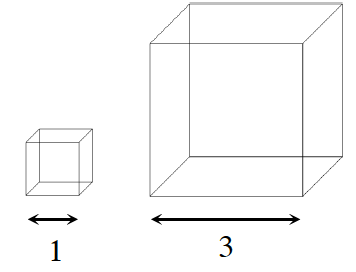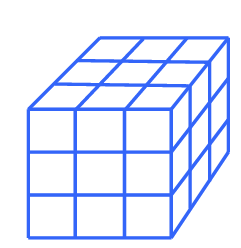### Home > GC > Chapter 8 > Lesson 8.3.3 > Problem8-111

8-111.

Multiple Choice: How many cubes with edge length $1$ unit would fit in a cube with edge length $3$ units?

1. $3$

1. $9$

1. $10$

1. $27$

1. None of theseDraw a rough sketch of a $3$ unit cube with $1$ unit cubes inside it.Multiply (length)(width)(height) to determine the correct answer.